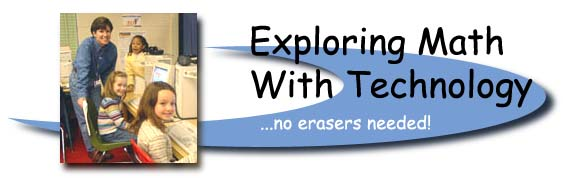Wicomico County Professional Day, January 30, 2004 | Interactive Math Resources | National Library of Virtual Manipulatives | Project Interactivate | | Math Zone (UK) | Ambleside Numeracy Page | NCTM E-Examples | McGraw-Hill Mathematics | | Primary Math Activities | Toftwood Numeracy Games | NCTM Illuminations Tools | | Kinsale First School Numeracy Resources | MD Voluntary State Curriculum | MSA Rubrics |
Primary Session

 Software Applications on our Network:

1. Kidspiration Activities - Math Standard: Money (K) 6A.3.a; (Gr. 1, 2) 6A.3.b; Fractions (Gr. 1, 2) 6A.2.a
2. See what's happening in the computer lab Examples of Math (and Language Arts) activities created by primary grade students. Math Standard: 1B.1.a - Kidspiration, KidPix, Windows Paint, Graph Club, MS Word, and Excel
3. Miss Damush's second grade students made graphs for an online project- "Graph Goodies"
4. Mrs. Hall's Kindergarten students use Graph Club. Ms. White's kindergarten students made graphs. Math Standard: (Gr. K, 1, 2) 4A.1.c
6. A digital quilt made by Mrs. Minor's kindergarten students using MS Windows Paint program. Math Standard: (K) 6A.1.e
7. KidPix and math - Math Standard: (K) 6A.1.g
 Online Interactive Math Websites:

Page numbers for BCRs refer to pages in the handouts for this session.

Standard 1.0 - Knowledge of Algebra, Patterns, or Functions

1. Pattern Blocks - Math Standard: (Gr. K, 1, 2) 1A.2.b Sample BCR (p. 1) and Sample BCR (p. 2) - Mrs. West's grade 1 students made patterns.
2. Pattern Blocks, Base Ten Blocks, and Integer Bars - examples of student work. Lesson plans
3. Complete the Pattern - Math Standard: (Gr. K, 1, 2) 1A.2.b
4. Splat Squares - an online 100 chart - Math Standard: (Gr. 1, 2) 6A.1.j; (Gr. 1, 2) 1A.1.a; (K) 6A.1.h - Sample BCR (p. 3)
5. Splat Squares - 100 chart without numbers, Math Standard: (Gr. 1, 2) 6A.1.j; (Gr. 1, 2) 1A.1.a; (K) 6A.1.h
6. Number chart up to 1000 and calculator. Math Standard: (Gr. 2) 6A.1.e
7. 100 grid - Math Standard: (Gr. 1, 2) 6A.1.k; (Gr. 1, 2) 1A.1.a; (K) 6A.1.h
8. Fishy Count - Math Standard: (K) 6A.1.g
9. Integer Bars - Math Standard: (Gr. K, 1, 2) 1B.1.a Sample BCR (p. 4)
10. Number Lines - Math Standard: (Gr. 1, 2) 1A.1.a Sample BCR (p. 5)
11. Missing number - with number line, Math Standard: (Gr. 2) 1B.2.b Sample BCR (p. 6)
12. Function Machine - Math Standard: (Gr. 2) 1A.1.c Sample BCR (p. 7)
13. Equivalence - Math Standard: (Gr. 1, 2) 1B.2 Sample BCR (p. 8) Sample BCR (p. 9)

Standard 3.0 - Knowledge of Measurement

1. Teaching Time - Click on "Whole Class Clock" and "Games" for each year. Math Standard: (Gr. 1, 2) 3A.1.b Sample BCR (p. 10)
2. Measuring inches - funbrain.com Math Standard: (Gr. 1, 2) 3B.1.a Sample BCR (p. 11)
3. Perimeter - Making rectangles with a Ladybug, Math Standard: (Gr. 2) 3C.1.a Sample BCR (p. 12)
4. Perimeter - Math Standard: (Gr. 2) 3C.1.a
5. USU Geoboard - Perimeter and Area, NCTM Geoboard Math Standard: (Gr. 2) 3C.1.a; Geometric Shapes (Gr. 1, 2) 2A.1.b (K) 2A.1.d Sample BCR (p. 13)
6. Area - Math Standard: (Gr. 2) 3C.1.b
7. Weight - to the nearest gram,(click "up to 500g" or "up to 1kg") Math Standard: (Gr. 2) 3B.1.c Sample BCR (p. 14)

Standard 4.0 - Knowledge of Statistics

1. Interpreting Data - Math Standard: (Gr. 1) 4A.1.b Sample BCR (p. 15)
2. Online Graph Maker - Math Standard: (Gr. 1, 2) 4A.1.d Sample BCR (p. 16)

Standard 6.0 - Knowledge of Number Relationships or Computation

1. Addition Machine - Math Standard: (Gr. 1, 2) 6C.1.a
2. Subtraction Machine Math Standard: (Gr. 1, 2) 6C.1.a
3. e-Manipulatives - Houghton-Mifflin: Money - Math Standard: (K) 6C.1.f; (Gr. K, 1, 2) 6A.3.a Sample BCR (p. 17)
4. e-Manipulatives - Houghton-Mifflin: Number Line - animal jumps from number to number - Math Standard: (K) 6A. 1.k
5. e-Manipulatives - Houghton-Mifflin: Counters Math Standard: (Gr. K, 1, 2) 6C.1.a

Standard 2.0 - Knowledge of Geometry

1. Hide the Ladybug - Math Standard: (Gr. 1, 2) 2D.1.a

 Online Global Projects at Delmar:

1. Mrs. Sandy Andrews and her grade 2 students - graphing with friends in Taiwan.
2. Mrs. Spicer's grade 1 students - and their friends in South Africa. From the teacher in South Africa: "Happy day (31 degrees forecast here - it's already stifling!!) Carolynn
3. Mrs. Claire Campbell's grade 1 students solve math puzzles sent by students in South Africa.
4. Mrs. Tardiff's kindergarten students made graphs of their favorite candy for online friends in Australia.
5. Delmar's Online Math Activities with friends around the world - Japan, South Africa, and Botswana
6. Non standard measurement - with friends in South Africa